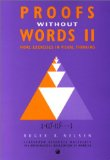# janmr blog

## Remembering Trigonometric Addition Formulas September 23, 2009

The addition formulas for sine and cosine look like this:

\begin{aligned} \cos(\alpha + \beta) &= \cos \alpha \cos \beta - \sin \alpha \sin \beta, \\ \sin(\alpha + \beta) &= \cos \alpha \sin \beta + \sin \alpha \cos \beta. \\ \end{aligned}

I can never remember them.

One solution is of course to look them up in a book or search the internet. What I usually do, though, is derive them using complex arithmetic. Apart from the usual rules known from real-valued arithmetic, all that is needed is knowing $e^{i \phi} = \cos \phi + i \sin \phi$ and $i^2 = -1$. Then you get

\begin{aligned} \cos(\alpha + \beta) + i \sin(\alpha + \beta) &= e^{i (\alpha+\beta)} \\ &= e^{i \alpha} e^{i \beta} \\ &= (\cos \alpha + i \sin \alpha)(\cos \beta + i \sin \beta) \\ &= (\cos \alpha \cos \beta - \sin \alpha \sin \beta) + i (\cos \alpha \sin \beta + \sin \alpha \cos \beta). \end{aligned}

By equating the real and imaginary parts you get the answer.

Flicking through Proofs Without Words II by Roger B. Nelsen, I saw the following wonderful figure. It could be a contender to an easier way to remember the addition formulas.

(Attributed to the author himself.) It should be pretty much self-explanatory. Apart from using sine and cosine to assign side-lengths to the four relevant right-angled triangles, all you need know is that the sum of the angles in a triangle is equal to two right angles (to realize that the two $\alpha$-angles are indeed equal).Both volumes of Proofs Without Words contain other “visual proofs” of the addition formulas. Some of these can also be found online.

How do you remember the addition formulas for sine and cosine?

Commenting is not possible for this post, but feel free to leave a question, correction or any comment by using the contact page